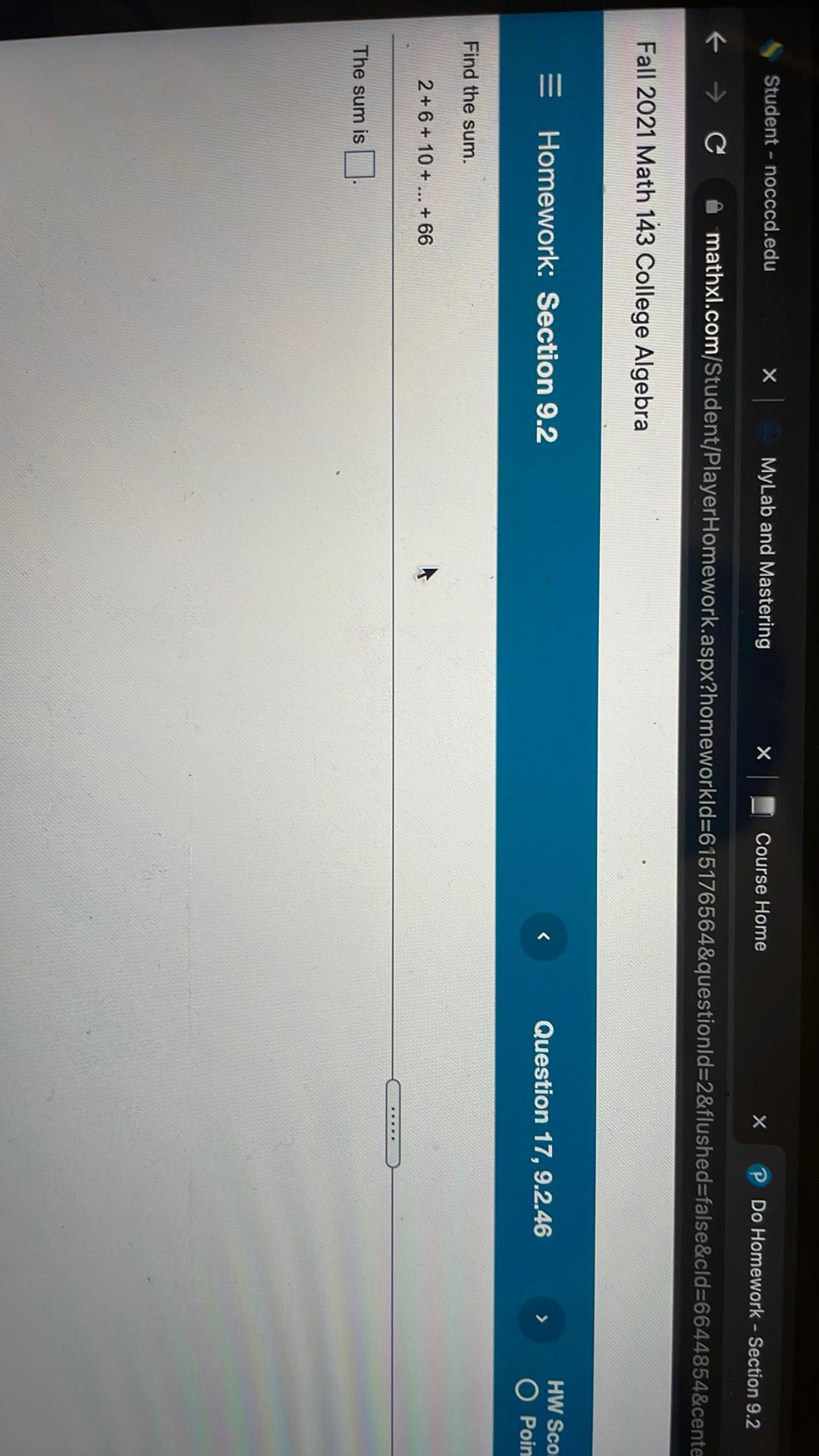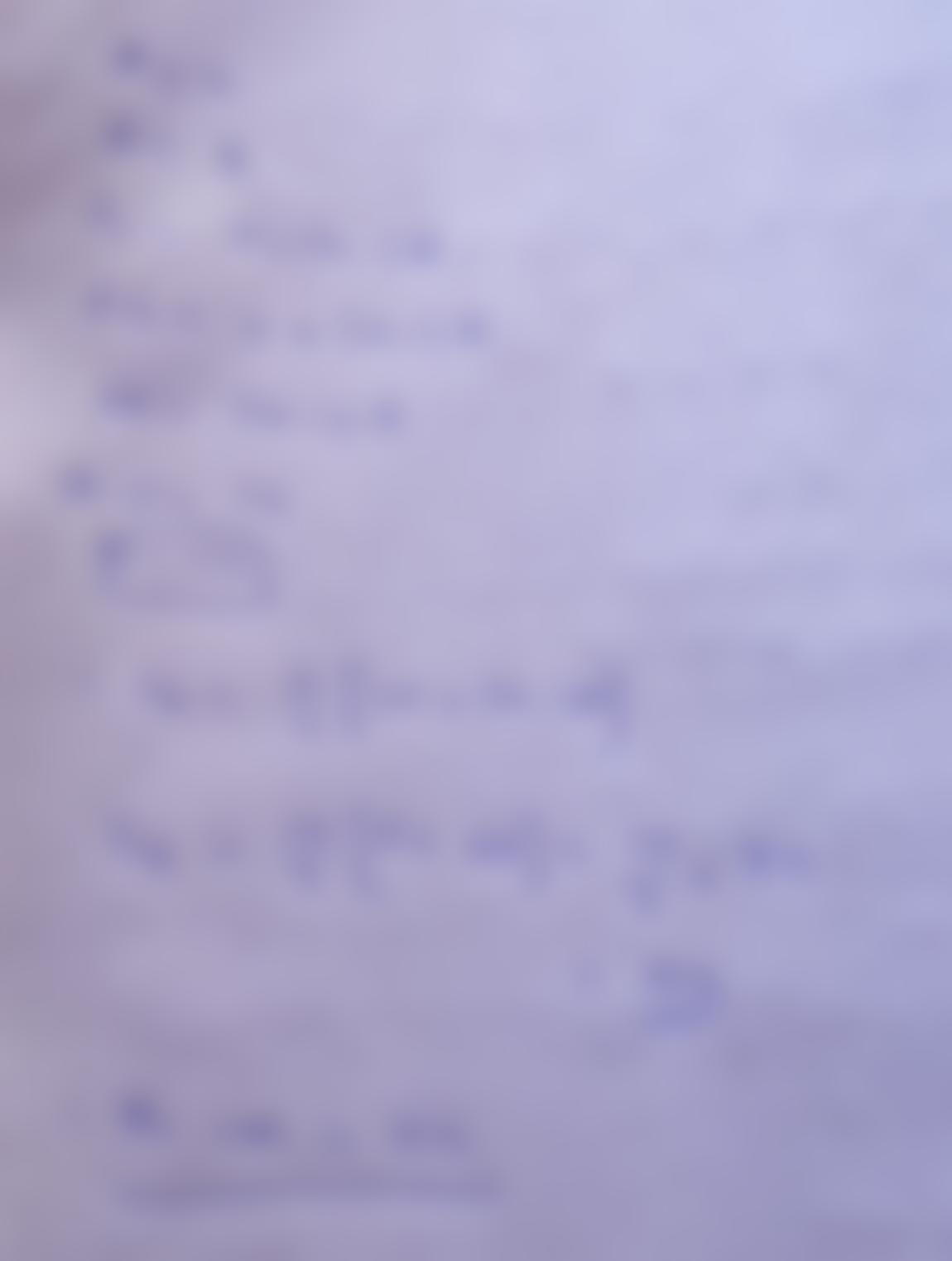Question:

Student - nocccd.edu x MyLab and Mastering x Course Home X Do Homework - Section 9.2 f → C mathxl.com/Student/PlayerHomework.aspStudent - nocccd.edu x MyLab and Mastering x Course Home X Do Homework - Section 9.2 f → C mathxl.com/Student/PlayerHomework.aspx?homeworkld=615176564&questionld=2&flushed=false&cld=6644854&cente Fall 2021 Math 143 College Algebra = Homework: Section 9.2 < Question 17, 9.2.46 HW Sco O Poin Find the sum. 2+ 6 + 10 + ... + 66 ..... The sum is